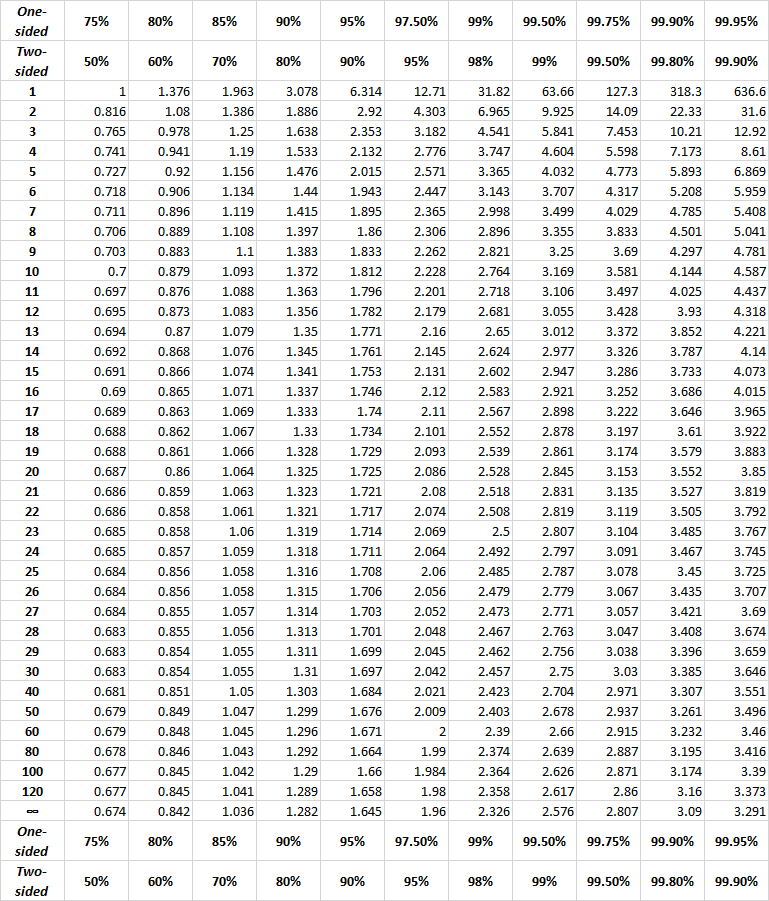Today May 12, 2023.......... 1.1K unique readers ...... 12K requests.......... official Cloudflare stats ..........

# The t-distribution table - how to use

#### How to use the t-tableAn experiment has 2 groups of subjects, 6 subjects in each group, 12 subjects total. Group 1 received a placebo (an inert substance). Group 2 received a drug. The temperature of each subject was recorded. We have 12 scores, 6 scores in each group.

Step 1. We calculate the means, mean 1 and mean 2.
Step 2. We calculate the degrees of freedom, df, is 10. How do we calculate df? df=total number of scores minus the number of means. In this case 12-2=10.
Step 3. We run the t-test for independent groups and, suppose, we find that t=4.52. We call this the calculated t.
Step 4. Now we go to the t-table and enter the left column at df 10
We now slide our finger to the right as far as column with the heading .........Next
Rate it:

### one-tail two tail

What is one-tail two-tail? How do I choose?

Rate it:### One-tail two-tail refers to

One-tail two-tail refers to the tails, the noses of the normal curve. In determining the p value, you may wish to consider weather what you measured in your experiment could vary up and down (two-tail) or only up or only down (one-tail). Examples: If in your experiment you are measuring height in teenagers as a result of a diet, you have a case of one-tail because you do not expect tanagers to grow shorter. If, however, you are measuring body weight, you have a case of two-tail, because tanagers may lose or gain body weight as a result of a diet.

Rate it:

### Look 95% two side at more

Look 95% two side at more than df 120. It is 1.96 .get it?

Rate it:

I don't get it,

Rate it:

p 0.05. Wow

Rate it:

### So, t distribution is

So, t distribution is adjusted for small samples?

Rate it:

### yes, for df, i.e. n

yes, for df, i.e. n

Rate it:

### Wow now I like stats!

Wow now I like stats!

Rate it: